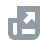Pay with
Markets
NFT
New
English
USDAccount FunctionsTutorialBinance Fan TokenBinance EarnCrypto Deposit/WithdrawalCrypto Derivatives
Futures Contracts
Introduction to Binance Futures
USDⓈ-M Futures Contracts
COIN-M Futures Contracts
Futures Guide
Cross Collateral
Hedge Mode
Types of Orders
Binance Futures Welcome Bonus Coupon
Futures Service Agreement
Binance Futures Related Programs
Index
Portfolio Margin
Options
Leveraged TokensFinanceAPISecurityOther TopicsNFTVIP

# How to Calculate Liquidation Price of Coin-M Futures Contracts

2021-02-05 07:59
Below is the liquidation price formula for Coin-M Futures contract:
where
Notes:
• In Cross Margin modeWB is crossWalletBalance
• In Isolated Margin mode, WB is Isolated Wallet Balance of the isolated position, TMM=0, UPNL=0, and substitutes the position quantity, MMR, cum into the formula to calculate.
• Under Cross Margin, the same ticker/symbol, both long and short position share the same liquidation price except in isolated mode. In Isolated Mode, each isolated position will have different liquidation prices depending on the margin allocated to the positions.
• If the liquidation price is less than 0, the UI display would be “--”

# Maintenance Margin Rate

You can find the “Maintenance Margin Rate” from Leverage and Margin in Coin-Margined Futures Contracts.
For example, if the position of the BTCUSD contract is 300 BTC, then the maintenance margin rate would be 12.5%.
Note:
If your position (calculated at the liquidation price) and the current position (calculated at the opening price) are of different levels, then you must substitute (i.e. to calculate at the liquidation price) the maintenance margin rate and the maintenance margin amount of the position level, to recalculate the liquidation price.

# Maintenance Amount

You can find the “Maintenance Amount” from the table below with the position value. For example, if the position of BTCUSD Contract is 300 BTC, then the maintenance amount would be 11.81 BTC.

## .css-yd6272{box-sizing:border-box;margin:0;min-width:0;color:#434343;}Maintenance Amount formula

Maintenance Amount = [Floor of Position Bracket on Level n * difference between Maintenance Margin Rate on Level n and Maintenance Margin Rate on Level n-1)] + Maintenance Amount on Level n-1
For example, the BTCUSD Maintenance Amount on Level 5
= 50 BTC*(5% -2.5%) + 0.56 BTC
= 1.81 BTC